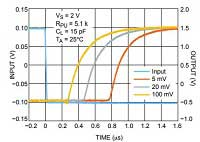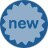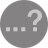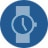# Why is my Comparator so Indecisive?Comparators seem pretty simple. They compare two signals voltages and set the output high or low accordingly. However, if both input signal voltages are really close, even a little noise on the input signal can cause the output to oscillate between high and low logic levels. Adding hysteresis is the easiest way around this problem.

Hysteresis is when the system output depends on its previous state. When hysteresis is added to a comparator, the upper switching threshold is set higher and the lower switching threshold is set lower. This is basically how an AC thermostat works, in case you haven’t noticed. Let’s take a moment to imagine if thermostats didn’t have hysteresis: the AC might cycle on and off every few seconds at the smallest temperature fluctuations, which would be noisy, inefficient, and put stress on the AC unit.  Adding hysteresis to the AC thermostat allows the system to operate more effectively.

Some comparators have built-in hysteresis, usually around a few millivolts. This may be adequate for certain applications, but other situations may require the addition of external hysteresis. Adding external hysteresis allows the rising and falling thresholds to be specific to the system requirements.

Hysteresis is implemented in comparator circuits through positive feedback. This is one of those few instances where positive feedback is a positive thing! Instead of having one threshold point, hysteresis creates different rising and falling thresholds. This keeps the output consistently at a low or high state instead of oscillating, even when the input signal is hovering around the reference voltage. A comparator with hysteresis added through positive feedback is also called a Schmitt trigger.

The example below shows the ON Semiconductor TL331 configured as an inverting Schmitt trigger. The TL331 is a single channel, low power, open collector comparator without internal hysteresis. The resistor divider created by the R1 and R2 resistors sets the reference voltage on the non-inverting pin, establishing the threshold voltage at which the comparator output switches. Since this is an open collector comparator, a pull-up resistor is connected to the output. The feedback resistor adds hysteresis through positive feedback. Typically, a relatively large feedback resistor value is used, at least 100 kΩ.Figure 1. Comparator configured as inverting Schmitt trigger

For this inverting configuration, when the input signal is low relative to the threshold, the output pin is high, pulling the threshold voltage higher through the feedback resistor. This way, small voltage fluctuations on the input signal don’t trigger the comparator output to switch until the input voltage reaches the higher, adjusted, rising threshold. Once the input signal reaches the rising threshold, the output is pulled low. This pulls the threshold voltage lower through the feedback resistor, so that the output will stay low until the input voltage drops to the below the lower adjusted threshold voltage.

The non-inverting configuration works in a similar way as far its use of positive feedback. However, in this case, the threshold voltage set by the resistor divider doesn’t change as it does with the inverting configuration. Instead, feedback adjusts the input signal at the non-inverting node.Figure 2. Comparator configured as non-inverting Schmitt trigger

In this configuration, when the input signal is low, the output pulls low, causing the voltage at the non-inverting node to drop lower. Once the input signal is high enough to pull the non-inverting node higher than the reference voltage, the output is pulled high, which then pulls the non-inverting node even higher.

In both circuits shown, adding hysteresis takes only one or two external resistors, and the resistor values can be adjusted to the threshold values best suited for the specific application. When designing in a comparator, if there is any chance that the voltages on the input pins will approach each other for any significant amount of time, adding hysteresis is a simple way of reducing issues caused by noise on the input signal..blogSocial img{ width: 30px; }7 2 0 0 1

Name*

Email*

Comment

Rules for CommentingConnect with us